# Algebra - problems - page 101

1. Supplementary angles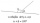One of the supplementary angles is larger by 33° than the second one. Calculate the angles size.
2. Non linear eqsSolve the system of non-linear equations: 3x2-3x-y=-2 -6x2-x-y=-7
3. Poplar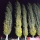How tall is a poplar by the river, if we know that 1/5 of its total height is a trunk, 1/10th of the height is the root and 35m from the trunk to the top of the poplar?
4. The lengthThe length of a rectangle is 6 meters less than twice the width. If the area of the rectangle is 216 meters, find the dimensions of the rectangle.
5. Evaluation of expressionsIf a2-3a+1=0, find (i)a2+1/a2 (ii) a3+1/a3
6. Three workshopsThere are 2743 people working in three workshops. In the second workshop works 140 people more than in the first and in third works 4.2 times more than the second one. How many people work in each workshop?
7. Account operationsMy savings of php 90,000 in a bank earns 6% interest in a year. If i will deposit additional php 10,000 at the end of 6 months, how much money will be left if i withdraw php 25,000 after a year?
8. Dog price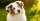Tereza agreed to get a dog and 6000 crowns a year for help in a dachshund breeding station. After eight months she had to finish work and got a dog and 2000 crowns. What price does a dog have?
9. TogetherIf 8 men, 10 women, 16 children collects ₹1024 in 4 days, how many days will be required for 6 men, 5 women and 4 boys to collect ₹768? (₹ is Indian Rupee)
10. Alice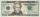Alice spent 5/11 of her money on a back pack. She has \$42 dollars left. How much was her back pack?
11. 12 apples12 apples and 2 loaves of bread cost 5.76 and 6 apples and 3 loaves of bread cost 7.68. How much is a loaf of bread?
12. HectolitersHow deep is the pool if there are 2025 hectoliters of water and the bottom dimensions are a = 15 meters b = 7,5 meters and the water level is up to 9/10 (nine-tenths) of height.
13. 600 pencils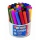600 pencils we want to be divided into three groups. The biggest groups have ten pens more than the smallest. How many ways can this be done?
14. Mini-surveyIn the mini-survey of our class about the popularity of individual subjects, it turned out that 11.1% of pupils like mathematics, 18.5% are enjoying languages, 30.4% of pupils like physical education, and the remaining 12 pupils have several popular subjec
15. Investment1000\$ is invested at 10% compound interest. What factor is the capital multiplied by each year? How much will be there after n=12 years?
16. Concentric circlesThere is given a circle K with a radius r = 8 cm. How large must a radius have a smaller concentric circle that divides the circle K into two parts with the same area?
17. PotatoesFor three days the store sold 1400 kg of potatoes. The first day they sold 100 kilograms of potatoes less than the second day, the third-day three-fifths of what they sold the first day. How many kgs of potatoes sold every day?
18. 40% volume40% volume with 104 uph (units per labor hour) 8 people working. What is the volume?
19. Perimeter of a rectangleIf the perimeter of a rectangle is 114 meters and the length is twice the width plus 6 meters, what are the length and width?
20. Centroid - two bodiesA body is composed of a 0.8 m long bar and a sphere with a radius of 0.1m attached so that its center lies on the longitudinal axis of the bar. Both bodies are of the same uniform material. The sphere is twice as heavy as the bar. Find the center of gravit

Do you have an interesting mathematical problem that you can't solve it? Enter it, and we can try to solve it.

To this e-mail address, we will reply solution; solved examples are also published here. Please enter e-mail correctly and check whether you don't have a full mailbox.